Theory and Modern Applications

# Positive solutions for second order impulsive differential equations with Stieltjes integral boundary conditions

## Abstract

In this paper, we study the existence of positive solutions for a singular second order impulsive differential equations with Stieltjes integral boundary conditions. By means of fixed point theorems, some results on the existence and multiplicity of positive solutions are obtained. Two examples are given to demonstrate the main results.

MSC:34B10, 34B15, 34B18, 34B37.

## 1 Introduction

In this paper, we consider the existence of positive solutions for the following second-order impulsive boundary value problem (IBVP for short)

$\left\{\begin{array}{c}{x}^{″}\left(t\right)+a\left(t\right){x}^{\prime }\left(t\right)+b\left(t\right)x\left(t\right)+h\left(t\right)f\left(t,x\left(t\right)\right)=0,\phantom{\rule{1em}{0ex}}t\in {J}^{\prime },\hfill \\ -\mathrm{△}{x}^{\prime }{|}_{t={t}_{i}}={I}_{i}\left(x\left({t}_{i}\right)\right),\phantom{\rule{1em}{0ex}}i=1,2,\dots ,m,\hfill \\ x\left(0\right)=\alpha \left[x\right],\phantom{\rule{2em}{0ex}}x\left(1\right)=\beta \left[x\right],\hfill \end{array}$
(1.1)

where $J=\left[0,1\right]$, $0<{t}_{1}<{t}_{2}<\cdots <{t}_{m}<1$, ${J}^{\prime }=J\setminus \left\{{t}_{1},{t}_{2},\dots ,{t}_{m}\right\}$, ${J}_{0}=\left(0,{t}_{1}\right],{J}_{1}=\left({t}_{1},{t}_{2}\right],\dots ,{J}_{m}=\left({t}_{m},1\right)$, $f\in C\left(J×{\mathbb{R}}^{+},{\mathbb{R}}^{+}\right)$, ${I}_{i}\in C\left({\mathbb{R}}^{+},{\mathbb{R}}^{+}\right)$, $i=1,2,\dots ,m$, ${\mathbb{R}}^{+}=\left[0,+\mathrm{\infty }\right)$. $\mathrm{△}{x}^{\prime }{|}_{t={t}_{i}}$ denotes the jump of ${x}^{\prime }$ at $t={t}_{i}$, i.e.,

$\mathrm{△}{x}^{\prime }{|}_{t={t}_{i}}={x}^{\prime }\left({t}_{i}^{+}\right)-{x}^{\prime }\left({t}_{i}^{-}\right),$

where ${x}^{\prime }\left({t}_{i}^{+}\right)$ and ${x}^{\prime }\left({t}_{i}^{-}\right)$ represent the right-hand limit and the left-hand limit of ${x}^{\prime }$ at ${t}_{i}$, respectively. $\alpha \left[x\right]$, $\beta \left[x\right]$ are linear functionals on $C\left(I\right)$ given by

$\alpha \left[x\right]={\int }_{0}^{1}x\left(t\right)\phantom{\rule{0.2em}{0ex}}dA\left(t\right),\phantom{\rule{2em}{0ex}}\beta \left[x\right]={\int }_{0}^{1}x\left(t\right)\phantom{\rule{0.2em}{0ex}}dB\left(t\right)$

involving Stieltjes integrals with signed measures, that is, A, B are suitable functions of bounded variation.

Impulsive differential equations describe processes with sudden changes in their state at certain moments. The theory of impulse differential equations has been further developed significantly in recent years and has played a very important role in modern applied mathematical modeling of real world processes in physics, population dynamics, chemical technology, biotechnology and economics. For details, see  and references therein.

Recently, Feng and Xie  have dealt with the second order m-point boundary value problem with impulse effects

$\left\{\begin{array}{c}-{x}^{″}\left(t\right)=f\left(t,x\left(t\right)\right),\phantom{\rule{1em}{0ex}}t\in J,\phantom{\rule{0.25em}{0ex}}t\ne {t}_{i},\hfill \\ -\mathrm{△}{x}^{\prime }{|}_{t={t}_{i}}={I}_{i}\left(x\left({t}_{i}\right)\right),\phantom{\rule{1em}{0ex}}i=1,2,\dots ,n,\hfill \\ x\left(0\right)={\sum }_{i=1}^{m-2}{a}_{i}x\left({\xi }_{i}\right),\phantom{\rule{2em}{0ex}}x\left(1\right)={\sum }_{i=1}^{m-2}{b}_{i}x\left({\xi }_{i}\right),\hfill \end{array}$

where ${a}_{i},{b}_{i}\in \left(0,1\right)$, $0<{\xi }_{1}<{\xi }_{2}<\cdots <{\xi }_{m-2}<1$, ${\sum }_{i=1}^{m-2}{b}_{i}{\xi }_{i}<1$, ${\sum }_{i=1}^{m-2}{a}_{i}\left(1-{\xi }_{i}\right)<1$. The existence results of one and two positive solutions are obtained based on the fixed point theorems in a cone.

For the case of ${I}_{i}=0$, $i=1,2,\dots ,m$, one of the special cases of problem (1.1) is the following multi-point boundary value problem

$\left\{\begin{array}{c}{x}^{″}\left(t\right)+a\left(t\right){x}^{\prime }\left(t\right)+b\left(t\right)x\left(t\right)+h\left(t\right)f\left(x\left(t\right)\right)=0,\phantom{\rule{1em}{0ex}}t\in \left(0,1\right),\hfill \\ x\left(0\right)=0,\phantom{\rule{2em}{0ex}}x\left(1\right)={\sum }_{i=1}^{m-2}{a}_{i}x\left({\xi }_{i}\right),\hfill \end{array}$
(1.2)

where $0<{\xi }_{1}<{\xi }_{2}<\cdots <{\xi }_{m-2}<1$. Boundary value problem (1.2) and related problems have been extensively studied in many papers in recent years (see  and references therein). The existence and multiplicity results of positive solutions are obtained by applying the Krasnosel’skii fixed-point theorem in cones, the Leggett-Williams fixed point theorem and the fixed point index theory. For example, Ma and Wang in  studied the existence of positive solutions to the nonlinear boundary-value problem

$\left\{\begin{array}{c}{x}^{″}\left(t\right)+a\left(t\right){x}^{\prime }\left(t\right)+b\left(t\right)x\left(t\right)+h\left(t\right)f\left(x\left(t\right)\right)=0,\phantom{\rule{1em}{0ex}}t\in \left(0,1\right),\hfill \\ x\left(0\right)=0,\phantom{\rule{2em}{0ex}}x\left(1\right)=\alpha x\left(\eta \right),\hfill \end{array}$
(1.3)

where $a\in C\left(J\right)$, $b\in C\left(J,\left(-\mathrm{\infty },0\right)\right)$, $0<\eta <1$ and $0<\alpha \varphi \left(\eta \right)<1$ are given, ϕ is the unique solution of the linear boundary value problem

$\left\{\begin{array}{c}{x}^{″}\left(t\right)+a\left(t\right){x}^{\prime }\left(t\right)+b\left(t\right)x\left(t\right)=0,\phantom{\rule{1em}{0ex}}t\in \left(0,1\right),\hfill \\ x\left(0\right)=0,\phantom{\rule{2em}{0ex}}x\left(1\right)=1.\hfill \end{array}$

The authors established the existence of at least one positive solution of (1.3) if f is either superlinear or sublinear by applying the fixed point theorem in cones.

Inspired by the work of the above papers, the aim of this paper is to establish the existence and multiplicity of positive solutions for the IBVP (1.1). We discuss the boundary value problem with Stieltjes integral boundary conditions, i.e., the IBVP (1.1) which includes second order two-point, three-point, multi-point and nonlocal boundary value problems as special cases. Moreover, $\alpha \left[\cdot \right]$ and $\beta \left[\cdot \right]$ are two linear functions on $C\left[0,1\right]$ denoting the Stieltjes integrals, where $A,B$ are of bounded variation, that is dA and dB may change sign. By using the Krasnosel’skii fixed-point theorem and the Leggett-Williams fixed point theorem, some existence and multiplicity results of positive solutions are obtained.

This paper is organized as follows. In Section 2, we present some preliminaries and lemmas. Section 3 is devoted to the proof of the main results. In Section 4, two examples are given to demonstrate the validity of our main results.

## 2 Some preliminaries and lemmas

In this section, we first introduce some background definitions in a Banach space, present some basic lemmas, and then present the fixed point theorems that are to be used in the proof of the main results.

Let with the norm ${\parallel x\parallel }_{P{C}^{1}}=max\left\{{\parallel x\parallel }_{PC},{\parallel {x}^{\prime }\parallel }_{PC}\right\}$, where

${\parallel x\parallel }_{PC}=\underset{t\in J}{sup}|x\left(t\right)|,\phantom{\rule{2em}{0ex}}{\parallel {x}^{\prime }\parallel }_{PC}=\underset{t\in J}{sup}|{x}^{\prime }\left(t\right)|.$

Then $P{C}^{1}\left(J,{\mathbb{R}}^{+}\right)$ is a Banach space. A function $x\in P{C}^{1}\left(J,{\mathbb{R}}^{+}\right)\cap {C}^{2}\left({J}^{\prime },\mathbb{R}\right)$ is called a positive solution of problem (1.1) if it satisfies (1.1).

Lemma 2.1 

Assume that $a\in C\left(J\right)$, $b\in C\left(J,\left(-\mathrm{\infty },0\right)\right)$. Let ϕ and ψ be the unique solution of the following boundary value problem

$\left\{\begin{array}{c}{\varphi }^{″}\left(t\right)+a\left(t\right){\varphi }^{\prime }\left(t\right)+b\left(t\right)\varphi \left(t\right)=0,\phantom{\rule{1em}{0ex}}t\in J,\hfill \\ \varphi \left(0\right)=0,\phantom{\rule{2em}{0ex}}\varphi \left(1\right)=1\hfill \end{array}$

and

$\left\{\begin{array}{c}{\psi }^{″}\left(t\right)+a\left(t\right){\psi }^{\prime }\left(t\right)+b\left(t\right)\psi \left(t\right)=0,\phantom{\rule{1em}{0ex}}t\in J,\hfill \\ \psi \left(0\right)=1,\phantom{\rule{2em}{0ex}}\psi \left(1\right)=0,\hfill \end{array}$

respectively. Then ϕ is strictly increasing on J, ψ is strictly decreasing on J.

Throughout this paper, we adopt the following assumptions:

(${H}_{1}$) $a\in C\left(J\right)$, $b\in C\left(J,\left(-\mathrm{\infty },0\right)\right)$, ${k}_{1},{k}_{4}\in \left(0,1\right]$, ${k}_{2},{k}_{3}\ge 0$, $k>0$, and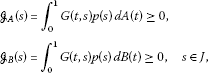where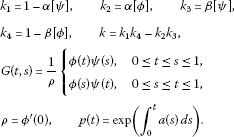(${H}_{2}$) $h:\left(0,1\right)\to {\mathbb{R}}^{+}$ is a Lebesgue integral and $0<{\int }_{0}^{1}h\left(t\right)\phantom{\rule{0.2em}{0ex}}dt<+\mathrm{\infty }$, ${I}_{i}:{\mathbb{R}}^{+}\to {\mathbb{R}}^{+}$ is continuous for $i=1,2,\dots ,m$.

(${H}_{3}$) $f:J×{\mathbb{R}}^{+}\to {\mathbb{R}}^{+}$ is continuous.

Remark 2.1 If dA and dB are two positive measures, then assumption (${H}_{1}$) can be replaced by the weaker assumption

(${H}_{1}^{\prime }$) $a\in C\left(J\right)$, $b\in C\left(J,\left(-\mathrm{\infty },0\right)\right)$, and ${k}_{1}>0$, ${k}_{4}>0$, $k>0$.

Lemma 2.2 Assume that (${H}_{1}$) holds. Then for any $y\in {L}^{1}\left[0,1\right]$, the problem

$\left\{\begin{array}{c}{x}^{″}\left(t\right)+a\left(t\right){x}^{\prime }\left(t\right)+b\left(t\right)x\left(t\right)+y\left(t\right)=0,\phantom{\rule{1em}{0ex}}t\in J,t\ne {t}_{i},\hfill \\ -\mathrm{△}{x}^{\prime }{|}_{t={t}_{i}}={I}_{i}\in \mathbb{R},\phantom{\rule{1em}{0ex}}i=1,2,\dots ,m,\hfill \\ x\left(0\right)=\alpha \left[x\right],\phantom{\rule{2em}{0ex}}x\left(1\right)=\beta \left[x\right],\hfill \end{array}$
(2.1)

has a unique solution given by the following formula:

$\begin{array}{rcl}x\left(t\right)& =& {\int }_{0}^{1}G\left(t,s\right)p\left(s\right)y\left(s\right)\phantom{\rule{0.2em}{0ex}}ds+\psi \left(t\right)\alpha \left[x\right]+\varphi \left(t\right)\beta \left[x\right]\\ +\psi \left(t\right)\sum _{i=1}^{j}{\rho }^{-1}p\left({t}_{i}\right)\varphi \left({t}_{i}\right){I}_{i}+\varphi \left(t\right)\sum _{i=j+1}^{m}{\rho }^{-1}p\left({t}_{i}\right)\psi \left({t}_{i}\right){I}_{i}\end{array}$
(2.2)

for $t\in {J}_{i}$, $i=0,1,\dots ,m$. Moreover, $x\left(t\right)\ge 0$ on J provided $y\ge 0$.

Proof By similar arguments in . So it is omitted. □

Remark 2.2 If (${H}_{1}$) holds, then for any $t,s\in J$, it is easy to testify that

$\gamma \left(t\right)G\left(s,s\right)\le G\left(t,s\right)\le G\left(s,s\right),$
(2.3)

where $\gamma \left(t\right)=min\left\{\varphi \left(t\right),\psi \left(t\right)\right\}$, $t\in J$. Let $\xi \in \left(0,{t}_{1}\right)$, $\eta \in \left({t}_{m},1\right)$, then

$G\left(t,s\right)\ge \gamma G\left(s,s\right),\phantom{\rule{1em}{0ex}}t\in \left[\xi ,\eta \right],s\in J,$
(2.4)

where $\gamma ={min}_{\xi \le t\le \eta }\gamma \left(t\right)$.

Put

$K=\left\{x\in PC\left(J,{\mathbb{R}}^{+}\right):\underset{\xi \le t\le \eta }{min}x\left(t\right)\ge \gamma {\parallel x\parallel }_{PC},\alpha \left[x\right]\ge 0,\beta \left[x\right]\ge 0\right\}.$

Clearly, K is a cone of $PC\left(J,{\mathbb{R}}^{+}\right)$. For any $r>0$, let ${K}_{r}=\left\{x\in K:{\parallel x\parallel }_{PC}, $\partial {K}_{r}=\left\{x\in K:{\parallel x\parallel }_{PC}=r\right\}$ and ${\overline{K}}_{r}=\left\{x\in K:{\parallel x\parallel }_{PC}\le r\right\}$.

For $x\in C\left(J,{\mathbb{R}}^{+}\right)$, we define two operators T and S by

$Tx\left(t\right)=\psi \left(t\right)\alpha \left[x\right]+\varphi \left(t\right)\beta \left[x\right]+Fx\left(t\right)+Qx\left(t\right)$

and

$\begin{array}{rcl}Sx\left(t\right)& =& {k}^{-1}\left({k}_{3}\varphi \left(t\right)+{k}_{4}\psi \left(t\right)\right)\left(\alpha \left[Fx\right]+\alpha \left[Qx\right]\right)\\ +{k}^{-1}\left({k}_{1}\varphi \left(t\right)+{k}_{2}\psi \left(t\right)\right)\left(\beta \left[Fx\right]+\beta \left[Qx\right]\right)+Fx\left(t\right)+Qx\left(t\right),\end{array}$

where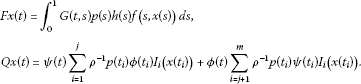Lemma 2.3 Assume that (${H}_{1}$)-(${H}_{3}$) hold. Then $T:K\to K$, $S:C\left(I,{\mathbb{R}}^{+}\right)\to K$ are completely continuous.

Proof Problem (1.1) has a solution x if and only if x solves the operator equation $x=Tx$ in K. Let $x\in K$, by (2.3) and the monotonicity of ϕ, ψ, we have

$\begin{array}{rcl}{\parallel Tx\parallel }_{PC}& \le & \alpha \left[x\right]+\beta \left[x\right]+{\int }_{0}^{1}G\left(s,s\right)p\left(s\right)h\left(s\right)f\left(s,x\left(s\right)\right)\phantom{\rule{0.2em}{0ex}}ds\\ +\sum _{i=1}^{j}{\rho }^{-1}p\left({t}_{i}\right)\varphi \left({t}_{i}\right){I}_{i}\left(x\left({t}_{i}\right)\right)+\sum _{i=j+1}^{m}{\rho }^{-1}p\left({t}_{i}\right)\psi \left({t}_{i}\right){I}_{i}\left(x\left({t}_{i}\right)\right).\end{array}$

Moreover, by (2.3) and the definition of γ, we have

$\begin{array}{rcl}\underset{\xi \le t\le \eta }{min}|Tx\left(t\right)|& \ge & \gamma \left(t\right)\alpha \left[x\right]+\gamma \left(t\right)\beta \left[x\right]+\gamma \left(t\right){\int }_{0}^{1}G\left(s,s\right)p\left(s\right)h\left(s\right)f\left(s,x\left(s\right)\right)\phantom{\rule{0.2em}{0ex}}ds\\ +\gamma \left(t\right)\sum _{i=1}^{j}{\rho }^{-1}p\left({t}_{i}\right)\varphi \left({t}_{i}\right){I}_{i}\left(x\left({t}_{i}\right)\right)+\gamma \left(t\right)\sum _{i=j+1}^{m}{\rho }^{-1}p\left({t}_{i}\right)\psi \left({t}_{i}\right){I}_{i}\left(x\left({t}_{i}\right)\right)\\ \ge & \gamma {\parallel Tx\parallel }_{PC}.\end{array}$

On the other hand,

$\begin{array}{rcl}\alpha \left[Tx\right]& =& \alpha \left[\psi \right]\alpha \left[x\right]+\alpha \left[\varphi \right]\beta \left[x\right]+\alpha \left[Fx\right]+\alpha \left[Qx\right]\\ =& \left(1-{k}_{1}\right)\alpha \left[x\right]+{k}_{2}\beta \left[x\right]+{\int }_{0}^{1}\left({\int }_{0}^{1}G\left(t,s\right)p\left(s\right)h\left(s\right)f\left(s,x\left(s\right)\right)\phantom{\rule{0.2em}{0ex}}ds\right)\phantom{\rule{0.2em}{0ex}}dA\left(t\right)\\ +\left(1-{k}_{1}\right)\sum _{i=1}^{j}{\rho }^{-1}p\left({t}_{i}\right)\varphi \left({t}_{i}\right){I}_{i}\left(x\left({t}_{i}\right)\right)+{k}_{2}\sum _{i=j+1}^{m}{\rho }^{-1}p\left({t}_{i}\right)\psi \left({t}_{i}\right){I}_{i}\left(x\left({t}_{i}\right)\right)\\ =& \left(1-{k}_{1}\right)\alpha \left[x\right]+{k}_{2}\beta \left[x\right]+{\int }_{0}^{1}{\mathcal{G}}_{A}\left(s\right)h\left(s\right)f\left(s,x\left(s\right)\right)\phantom{\rule{0.2em}{0ex}}ds\\ +\left(1-{k}_{1}\right)\sum _{i=1}^{j}{\rho }^{-1}p\left({t}_{i}\right)\varphi \left({t}_{i}\right){I}_{i}\left(x\left({t}_{i}\right)\right)+{k}_{2}\sum _{i=j+1}^{m}{\rho }^{-1}p\left({t}_{i}\right)\psi \left({t}_{i}\right){I}_{i}\left(x\left({t}_{i}\right)\right)\ge 0,\\ \beta \left[Tx\right]& =& \beta \left[\psi \right]\alpha \left[x\right]+\beta \left[\varphi \right]\beta \left[x\right]+\beta \left[Fx\right]+\beta \left[Qx\right]\\ =& {k}_{3}\alpha \left[x\right]+\left(1-{k}_{4}\right)\beta \left[x\right]+{\int }_{0}^{1}\left({\int }_{0}^{1}G\left(t,s\right)p\left(s\right)h\left(s\right)f\left(s,x\left(s\right)\right)\phantom{\rule{0.2em}{0ex}}ds\right)\phantom{\rule{0.2em}{0ex}}dB\left(t\right)\\ +{k}_{3}\sum _{i=1}^{j}{\rho }^{-1}p\left({t}_{i}\right)\varphi \left({t}_{i}\right){I}_{i}\left(x\left({t}_{i}\right)\right)+\left(1-{k}_{4}\right)\sum _{i=j+1}^{m}{\rho }^{-1}p\left({t}_{i}\right)\psi \left({t}_{i}\right){I}_{i}\left(x\left({t}_{i}\right)\right)\\ =& {k}_{3}\alpha \left[x\right]+\left(1-{k}_{4}\right)\beta \left[x\right]+{\int }_{0}^{1}{\mathcal{G}}_{B}\left(s\right)h\left(s\right)f\left(s,x\left(s\right)\right)\phantom{\rule{0.2em}{0ex}}ds\\ +{k}_{3}\sum _{i=1}^{j}{\rho }^{-1}p\left({t}_{i}\right)\varphi \left({t}_{i}\right){I}_{i}\left(x\left({t}_{i}\right)\right)+\left(1-{k}_{4}\right)\sum _{i=j+1}^{m}{\rho }^{-1}p\left({t}_{i}\right)\psi \left({t}_{i}\right){I}_{i}\left(x\left({t}_{i}\right)\right)\ge 0.\end{array}$

This shows that $T:K\to K$.

Now we consider the operator S. Similarly as for the operator T, we have

$\begin{array}{rcl}{\parallel Sx\parallel }_{PC}& \le & {k}^{-1}\left({k}_{3}+{k}_{4}\right)\left(\alpha \left[Fx\right]+\alpha \left[Qx\right]\right)+{k}^{-1}\left({k}_{1}+{k}_{2}\right)\left(\beta \left[Fx\right]+\beta \left[Qx\right]\right)\\ +{\int }_{0}^{1}G\left(s,s\right)p\left(s\right)h\left(s\right)f\left(s,x\left(s\right)\right)\phantom{\rule{0.2em}{0ex}}ds\\ +\sum _{i=1}^{j}{\rho }^{-1}p\left({t}_{i}\right)\varphi \left({t}_{i}\right){I}_{i}\left(x\left({t}_{i}\right)\right)+\sum _{i=j+1}^{m}{\rho }^{-1}p\left({t}_{i}\right)\psi \left({t}_{i}\right){I}_{i}\left(x\left({t}_{i}\right)\right),\end{array}$

and

$\begin{array}{rcl}\underset{\xi \le t\le \eta }{min}|Sx\left(t\right)|& \ge & {k}^{-1}\left({k}_{3}+{k}_{4}\right)\gamma \left(t\right)\left(\alpha \left[Fx\right]+\alpha \left[Qx\right]\right)+{k}^{-1}\left({k}_{1}+{k}_{2}\right)\gamma \left(t\right)\left(\beta \left[Fx\right]+\beta \left[Qx\right]\right)\\ +\gamma \left(t\right){\int }_{0}^{1}G\left(s,s\right)p\left(s\right)h\left(s\right)f\left(s,x\left(s\right)\right)\phantom{\rule{0.2em}{0ex}}ds\\ +\gamma \left(t\right)\sum _{i=1}^{j}{\rho }^{-1}p\left({t}_{i}\right)\varphi \left({t}_{i}\right){I}_{i}\left(x\left({t}_{i}\right)\right)+\gamma \left(t\right)\sum _{i=j+1}^{m}{\rho }^{-1}p\left({t}_{i}\right)\psi \left({t}_{i}\right){I}_{i}\left(x\left({t}_{i}\right)\right)\\ \ge & \gamma {\parallel Sx\parallel }_{PC}.\end{array}$

Moreover,

$\begin{array}{rcl}\alpha \left[Sx\right]& =& {k}^{-1}\left({k}_{3}{k}_{2}+{k}_{4}\left(1-{k}_{1}\right)\right)\left(\alpha \left[Fx\right]+\alpha \left[Qx\right]\right)\\ +{k}^{-1}\left({k}_{1}{k}_{2}+{k}_{2}\left(1-{k}_{1}\right)\right)\left(\beta \left[Fx\right]+\beta \left[Qx\right]\right)+\alpha \left[Fx\right]+\alpha \left[Qx\right]\\ =& {k}^{-1}{k}_{4}\left(\alpha \left[Fx\right]+\alpha \left[Qx\right]\right)+{k}^{-1}{k}_{2}\left(\beta \left[Fx\right]+\beta \left[Qx\right]\right)\ge 0,\end{array}$
(2.5)
$\begin{array}{rcl}\beta \left[Sx\right]& =& {k}^{-1}\left({k}_{3}\left(1-{k}_{4}\right)+{k}_{4}{k}_{3}\right)\left(\alpha \left[Fx\right]+\alpha \left[Qx\right]\right)\\ +{k}^{-1}\left({k}_{1}\left(1-{k}_{4}\right)+{k}_{2}{k}_{3}\right)\left(\beta \left[Fx\right]+\beta \left[Qx\right]\right)+\beta \left[Fx\right]+\beta \left[Qx\right]\\ =& {k}^{-1}{k}_{3}\left(\alpha \left[Fx\right]+\alpha \left[Qx\right]\right)+{k}^{-1}{k}_{1}\left(\beta \left[Fx\right]+\beta \left[Qx\right]\right)\ge 0.\end{array}$
(2.6)

This yields that $S:C\left(J,{\mathbb{R}}^{+}\right)\to K$.

Next, by similar arguments in , one can prove that $T:K\to K$, $S:C\left(J,{\mathbb{R}}^{+}\right)\to K$ are completely continuous. So we omit further details, and Lemma 2.3 is proved. □

Lemma 2.4 Assume that (${H}_{1}$)-(${H}_{3}$) hold. Then operators T and S have the same fixed point in K.

Proof Let x be a fixed point of the operator S, i.e., $x=Sx$. Then by (2.5) and (2.6), we have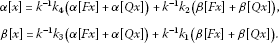So we have

$\alpha \left[Fx\right]+\alpha \left[Qx\right]={k}_{1}\alpha \left[x\right]-{k}_{2}\beta \left[x\right],\phantom{\rule{2em}{0ex}}\beta \left[Fx\right]+\beta \left[Qx\right]=-{k}_{3}\alpha \left[x\right]+{k}_{4}\beta \left[x\right].$

Then

$\begin{array}{rcl}x\left(t\right)& =& Sx\left(t\right)\\ =& {k}^{-1}\left({k}_{3}\varphi \left(t\right)+{k}_{4}\psi \left(t\right)\right)\left(\alpha \left[Fx\right]+\alpha \left[Qx\right]\right)\\ +{k}^{-1}\left({k}_{1}\varphi \left(t\right)+{k}_{2}\psi \left(t\right)\right)\left(\beta \left[Fx\right]+\beta \left[Qx\right]\right)+Fx\left(t\right)+Qx\left(t\right)\\ =& {k}^{-1}\left({k}_{3}\varphi \left(t\right)+{k}_{4}\psi \left(t\right)\right)\left({k}_{1}\alpha \left[x\right]-{k}_{2}\beta \left[x\right]\right)\\ +{k}^{-1}\left({k}_{1}\varphi \left(t\right)+{k}_{2}\psi \left(t\right)\right)\left(-{k}_{3}\alpha \left[x\right]+{k}_{4}\beta \left[x\right]\right)+Fx\left(t\right)+Qx\left(t\right)\\ =& \psi \left(t\right)\alpha \left[x\right]+\varphi \left(t\right)\beta \left[x\right]+Fx\left(t\right)+Qx\left(t\right)=Tx\left(t\right).\end{array}$

This implies that x also is a fixed point of the operator T.

On the other hand, let x be a fixed point of the operator T, i.e., $x=Tx$. Then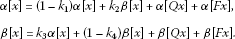So we have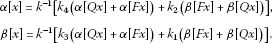Therefore,

$\begin{array}{rcl}x\left(t\right)& =& Tx\left(t\right)\\ =& \psi \left(t\right)\alpha \left[x\right]+\varphi \left(t\right)\beta \left[x\right]+Fx\left(t\right)+Qx\left(t\right)\\ =& \psi \left(t\right){k}^{-1}\left[{k}_{4}\left(\alpha \left[Qx\right]+\alpha \left[Fx\right]\right)+{k}_{2}\left(\beta \left[Fx\right]+\beta \left[Qx\right]\right)\right]\\ +\varphi \left(t\right){k}^{-1}\left[{k}_{3}\left(\alpha \left[Qx\right]+\alpha \left[Fx\right]\right)+{k}_{1}\left(\beta \left[Fx\right]+\beta \left[Qx\right]\right)\right]+Fx\left(t\right)+Qx\left(t\right)\\ =& {k}^{-1}\left({k}_{3}\varphi \left(t\right)+{k}_{4}\psi \left(t\right)\right)\left(\alpha \left[Fx\right]+\alpha \left[Qx\right]\right)\\ +{k}^{-1}\left({k}_{1}\varphi \left(t\right)+{k}_{2}\psi \left(t\right)\right)\left(\beta \left[Fx\right]+\beta \left[Qx\right]\right)+Fx\left(t\right)+Qx\left(t\right)=Sx\left(t\right).\end{array}$

This implies that x is also a fixed point of the operator S. The proof is completed. □

Lemma 2.5 

Let X be a real Banach space, K is a cone in X. Assume that ${\mathrm{\Omega }}_{1}$ and ${\mathrm{\Omega }}_{2}$ are two bounded open sets of X with $\theta \in {\mathrm{\Omega }}_{1}$ and ${\overline{\mathrm{\Omega }}}_{1}\subset {\mathrm{\Omega }}_{2}$. Let $T:K\cap \left({\overline{\mathrm{\Omega }}}_{2}\mathrm{\setminus }{\mathrm{\Omega }}_{1}\right)\to K$ be a completely continuous operator such that either

1. (i)

$\parallel Tx\parallel \le \parallel x\parallel$, $x\in K\cap \partial {\mathrm{\Omega }}_{1}$ and $\parallel Tx\parallel \ge \parallel x\parallel$, $x\in K\cap \partial {\mathrm{\Omega }}_{2}$, or

2. (ii)

$\parallel Tx\parallel \ge \parallel x\parallel$, $x\in K\cap \partial {\mathrm{\Omega }}_{1}$ and $\parallel Tx\parallel \le \parallel x\parallel$, $x\in K\cap \partial {\mathrm{\Omega }}_{2}$.

Then T has a fixed point in $K\cap \left({\overline{\mathrm{\Omega }}}_{2}\setminus {\mathrm{\Omega }}_{1}\right)$.

Lemma 2.6 [18, 19]

Let K be a cone in a real Banach space X, ${K}_{c}=\left\{x\in K:\parallel x\parallel , φ is a nonnegative continuous concave functional on K such that $\phi \left(x\right)\le \parallel x\parallel$, for all $x\in {\overline{K}}_{c}$, and $K\left(\phi ,b,d\right)=\left\{x\in K:b\le \phi \left(x\right),\parallel x\parallel \le d\right\}$. Suppose that $T:{\overline{K}}_{c}\to {\overline{K}}_{c}$ is completely continuous and there exist positive constants $0 such that

(${C}_{1}$) $\left\{x\in K\left(\phi ,b,d\right):\phi \left(x\right)>b\right\}\ne \varphi$ and $\phi \left(Tx\right)>b$ for $x\in K\left(\phi ,b,d\right)$,

(${C}_{2}$) $\parallel Tx\parallel for $x\in {\overline{K}}_{a}$,

(${C}_{3}$) $\phi \left(Tx\right)>b$ for $x\in K\left(\phi ,b,c\right)$ with $\parallel Tx\parallel >d$.

Then T has at least three fixed points ${x}_{1}$, ${x}_{2}$ and ${x}_{3}$ with

$\parallel {x}_{1}\parallel

Remark 2.3 If there holds $d=c$, then condition (${C}_{1}$) of Lemma 2.6 implies condition (${C}_{3}$) of Lemma 2.6.

## 3 Main results

Let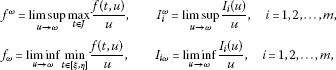where ω denotes 0 or ∞. Let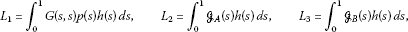(3.1)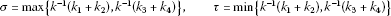(3.2)

and let the nonnegative continuous concave functional φ on the cone K be defined by

$\phi \left(x\right)=\underset{\xi \le t\le \eta }{min}|x\left(t\right)|.$

In this section, we apply Lemmas 2.5 and 2.6 to establish the existence of positive solutions for IBVP (1.1). Since operators T and S have the same fixed points (see Lemma 2.4), to prove the following theorems we always use the operator S instead of T.

Theorem 3.1 Assume that (${H}_{1}$)-(${H}_{3}$) hold. In addition, suppose ${f}^{0}+{\sum }_{j=1}^{m}{I}_{j}^{0}=0$ and $max\left\{{f}_{\mathrm{\infty }},{I}_{1\mathrm{\infty }},\dots ,{I}_{m\mathrm{\infty }}\right\}=\mathrm{\infty }$ are satisfied, then IBVP (1.1) has at least one positive solution ${x}^{\ast }\left(t\right)$.

Proof From ${f}^{0}+{\sum }_{j=1}^{m}{I}_{j}^{0}=0$, there exists ${r}_{1}>0$ such that $f\left(t,u\right)<{\epsilon }_{1}u$, ${I}_{j}\left(u\right)<{\epsilon }_{1}u$ ($j=1,2,\dots ,m$) for all $t\in J$ and $0\le u\le {r}_{1}$, where ${\epsilon }_{1}>0$ satisfies

${\epsilon }_{1}
(3.3)

If $x\in {\overline{K}}_{{r}_{1}}$, then ${\parallel x\parallel }_{PC}\le {r}_{1}$. So we have $0\le x\left(t\right)\le {r}_{1}$, $t\in J$ and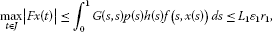(3.4)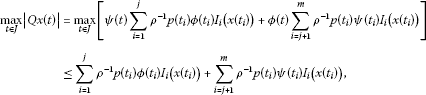(3.5)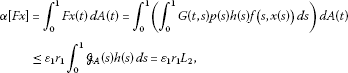(3.6)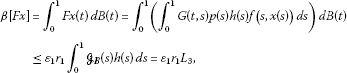(3.7)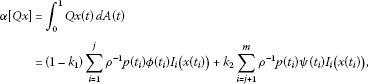(3.8)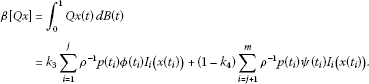(3.9)

So, by (3.4)-(3.9), for any $x\in \partial {K}_{{r}_{1}}$, $t\in J$, we have

$\begin{array}{rcl}|Sx\left(t\right)|& =& {k}^{-1}\left({k}_{3}\varphi \left(t\right)+{k}_{4}\psi \left(t\right)\right)\left(\alpha \left[Fx\right]+\alpha \left[Qx\right]\right)\\ +{k}^{-1}\left({k}_{1}\varphi \left(t\right)+{k}_{2}\psi \left(t\right)\right)\left(\beta \left[Fx\right]+\beta \left[Qx\right]\right)+Fx\left(t\right)+Qx\left(t\right)\\ \le & {k}^{-1}\left({k}_{3}+{k}_{4}\right)\left(\alpha \left[Fx\right]+\alpha \left[Qx\right]\right)+{k}^{-1}\left({k}_{1}+{k}_{2}\right)\left(\beta \left[Fx\right]+\beta \left[Qx\right]\right)\\ +\underset{t\in J}{max}|Fx\left(t\right)|+\underset{t\in J}{max}|Qx\left(t\right)|\\ \le & {k}^{-1}\left({k}_{3}+{k}_{4}\right)\alpha \left[Fx\right]+{k}^{-1}\left({k}_{1}+{k}_{2}\right)\beta \left[Fx\right]+\underset{t\in J}{max}|Fx\left(t\right)|\\ +{k}^{-1}\left({k}_{3}+{k}_{4}\right)\left(\left(1-{k}_{1}\right)\sum _{i=1}^{j}{\rho }^{-1}p\left({t}_{i}\right)\varphi \left({t}_{i}\right){I}_{i}\left(x\left({t}_{i}\right)\right)\\ +{k}_{2}\sum _{i=j+1}^{m}{\rho }^{-1}p\left({t}_{i}\right)\psi \left({t}_{i}\right){I}_{i}\left(x\left({t}_{i}\right)\right)\right)\\ +{k}^{-1}\left({k}_{1}+{k}_{2}\right)\left({k}_{3}\sum _{i=1}^{j}{\rho }^{-1}p\left({t}_{i}\right)\varphi \left({t}_{i}\right){I}_{i}\left(x\left({t}_{i}\right)\right)\\ +\left(1-{k}_{4}\right)\sum _{i=j+1}^{m}{\rho }^{-1}p\left({t}_{i}\right)\psi \left({t}_{i}\right){I}_{i}\left(x\left({t}_{i}\right)\right)\right)\\ +\sum _{i=1}^{j}{\rho }^{-1}p\left({t}_{i}\right)\varphi \left({t}_{i}\right){I}_{i}\left(x\left({t}_{i}\right)\right)+\sum _{i=j+1}^{m}{\rho }^{-1}p\left({t}_{i}\right)\psi \left({t}_{i}\right){I}_{i}\left(x\left({t}_{i}\right)\right)\\ \le & {k}^{-1}\left({k}_{3}+{k}_{4}\right){L}_{2}{\epsilon }_{1}{r}_{1}+{k}^{-1}\left({k}_{1}+{k}_{2}\right){L}_{3}{\epsilon }_{1}{r}_{1}+{L}_{1}{\epsilon }_{1}{r}_{1}\\ +{k}^{-1}\left({k}_{3}+{k}_{4}\right)\sum _{i=1}^{j}{\rho }^{-1}p\left({t}_{i}\right)\varphi \left({t}_{i}\right){I}_{i}\left(x\left({t}_{i}\right)\right)\\ +{k}^{-1}\left({k}_{1}+{k}_{2}\right)\sum _{i=j+1}^{m}{\rho }^{-1}p\left({t}_{i}\right)\psi \left({t}_{i}\right){I}_{i}\left(x\left({t}_{i}\right)\right)\\ \le & {\epsilon }_{1}\left[{L}_{1}+\sigma \left({L}_{2}+{L}_{3}\right)\right]{r}_{1}+{\epsilon }_{1}{r}_{1}\sigma \left[\sum _{i=1}^{j}{\rho }^{-1}p\left({t}_{i}\right)\varphi \left({t}_{i}\right)+\sum _{i=j+1}^{m}{\rho }^{-1}p\left({t}_{i}\right)\psi \left({t}_{i}\right)\right]\\ <& {r}_{1}={\parallel x\parallel }_{PC},\end{array}$
(3.10)

which means that

${\parallel Sx\parallel }_{PC}\le {\parallel x\parallel }_{PC},\phantom{\rule{1em}{0ex}}x\in \partial {K}_{{r}_{1}}.$
(3.11)

Next, consider $max\left\{{f}_{\mathrm{\infty }},{I}_{1\mathrm{\infty }},\dots ,{I}_{m\mathrm{\infty }}\right\}=\mathrm{\infty }$. Without loss of generality, we assume that $max\left\{{f}_{\mathrm{\infty }},{I}_{1\mathrm{\infty }},\dots ,{I}_{m\mathrm{\infty }}\right\}={f}_{\mathrm{\infty }}$, which means that there exists ${\overline{r}}_{2}>0$ such that $f\left(t,u\right)>{\epsilon }_{2}u$ for all $t\in \left[\xi ,\eta \right]$ and $u\ge {\overline{r}}_{2}$, where ${\epsilon }_{2}$ satisfies

${\epsilon }_{2}>max\left\{\frac{1}{\gamma \left[{L}_{1}+\tau \left({L}_{2}+{L}_{3}\right)\right]},\frac{1}{\gamma \tau \left({\sum }_{i=1}^{j}{\rho }^{-1}p\left({t}_{i}\right)\varphi \left({t}_{i}\right)+{\sum }_{i=j+1}^{m}{\rho }^{-1}p\left({t}_{i}\right)\psi \left({t}_{i}\right)\right)}\right\}.$
(3.12)

Let ${r}_{2}\ge max\left\{2{r}_{1},\frac{{\overline{r}}_{2}}{\gamma }\right\}$, then for any $x\in \partial {K}_{{r}_{2}}$, $t\in \left[\xi ,\eta \right]$, we have

$\begin{array}{rcl}|Sx\left(t\right)|& \ge & \underset{\xi \le t\le \eta }{min}\left({k}^{-1}\left({k}_{3}\varphi \left(t\right)+{k}_{4}\psi \left(t\right)\right)\left(\alpha \left[Fx\right]+\alpha \left[Qx\right]\right)\\ +{k}^{-1}\left({k}_{1}\varphi \left(t\right)+{k}_{2}\psi \left(t\right)\right)\left(\beta \left[Fx\right]+\beta \left[Qx\right]\right)+Fx\left(t\right)+Qx\left(t\right)\right)\\ \ge & \gamma {k}^{-1}\left({k}_{3}+{k}_{4}\right)\alpha \left[Fx\right]+\gamma {k}^{-1}\left({k}_{1}+{k}_{2}\right)\beta \left[Fx\right]\\ +\gamma {\int }_{0}^{1}G\left(s,s\right)p\left(s\right)h\left(s\right)f\left(s,x\left(s\right)\right)\phantom{\rule{0.2em}{0ex}}ds\\ \ge & \gamma \left[{k}^{-1}\left({k}_{3}+{k}_{4}\right){L}_{2}{\epsilon }_{2}{r}_{2}+{k}^{-1}\left({k}_{1}+{k}_{2}\right){L}_{3}{\epsilon }_{2}{r}_{2}+{L}_{1}{\epsilon }_{2}{r}_{2}\right]\\ \ge & \gamma {\epsilon }_{2}\left[{L}_{1}+\tau \left({L}_{2}+{L}_{3}\right)\right]{r}_{2}\\ >& {r}_{2}={\parallel x\parallel }_{PC}.\end{array}$

Consequently, we have

${\parallel Sx\parallel }_{PC}\ge {\parallel x\parallel }_{PC},\phantom{\rule{1em}{0ex}}x\in \partial {K}_{{r}_{2}}.$
(3.13)

Applying (i) of Lemma 2.5 to (3.11) and (3.13) yields that S has a fixed point ${x}^{\ast }:{r}_{1}\le {\parallel {x}^{\ast }\parallel }_{PC}\le {r}_{2}$. Thus it follows that IBVP (1.1) has a positive solution ${x}^{\ast }$. □

Theorem 3.2 Assume that (${H}_{1}$)-(${H}_{3}$) hold. In addition, suppose ${f}^{\mathrm{\infty }}+{\sum }_{j=1}^{m}{I}_{j}^{\mathrm{\infty }}=0$ and $max\left\{{f}_{0},{I}_{10},\dots ,{I}_{m0}\right\}=\mathrm{\infty }$ are satisfied, then IBVP (1.1) has at least one positive solution ${x}^{\ast }\left(t\right)$.

Proof Consider $max\left\{{f}_{0},{I}_{10},\dots ,{I}_{m0}\right\}=\mathrm{\infty }$. Without loss of generality, we assume that $max\left\{{f}_{0},{I}_{10},\dots ,{I}_{m0}\right\}={f}_{0}$, which means that there exists ${R}_{1}>0$ such that $f\left(t,u\right)>{\epsilon }_{2}u$ for all $t\in \left[\xi ,\eta \right]$ and $0\le u\le {R}_{1}$, where ${\epsilon }_{2}$ satisfies (3.12). Then for any $x\in \partial {K}_{{R}_{1}}$, $t\in \left[\xi ,\eta \right]$, we have

$\begin{array}{rcl}|Sx\left(t\right)|& \ge & \underset{\xi \le t\le \eta }{min}\left({k}^{-1}\left({k}_{3}\varphi \left(t\right)+{k}_{4}\psi \left(t\right)\right)\left(\alpha \left[Fx\right]+\alpha \left[Qx\right]\right)\\ +{k}^{-1}\left({k}_{1}\varphi \left(t\right)+{k}_{2}\psi \left(t\right)\right)\left(\beta \left[Fx\right]+\beta \left[Qx\right]\right)+Fx\left(t\right)+Qx\left(t\right)\right)\\ \ge & \gamma {k}^{-1}\left({k}_{3}+{k}_{4}\right)\alpha \left[Fx\right]+\gamma {k}^{-1}\left({k}_{1}+{k}_{2}\right)\beta \left[Fx\right]\\ +\gamma {\int }_{0}^{1}G\left(s,s\right)p\left(s\right)h\left(s\right)f\left(s,x\left(s\right)\right)\phantom{\rule{0.2em}{0ex}}ds\\ \ge & \gamma \left[{k}^{-1}\left({k}_{3}+{k}_{4}\right){L}_{2}{\epsilon }_{2}{R}_{1}+{k}^{-1}\left({k}_{1}+{k}_{2}\right){L}_{3}{\epsilon }_{2}{R}_{1}+{L}_{1}{\epsilon }_{2}{R}_{1}\right]\\ \ge & \gamma {\epsilon }_{2}\left[{L}_{1}+\tau \left({L}_{2}+{L}_{3}\right)\right]{r}_{2}\\ >& {R}_{1}={\parallel x\parallel }_{PC},\end{array}$

which yields that

${\parallel Sx\parallel }_{PC}\ge {\parallel x\parallel }_{PC},\phantom{\rule{1em}{0ex}}x\in \partial {K}_{{R}_{1}}.$
(3.14)

On the other hand, it follows from ${f}^{\mathrm{\infty }}+{\sum }_{j=1}^{m}{I}_{j}^{\mathrm{\infty }}=0$ that there exists ${R}_{2}:{R}_{2}>{R}_{1}$ such that $f\left(t,u\right)<{\epsilon }_{1}u$, ${I}_{j}\left(u\right)<{\epsilon }_{1}u$ ($j=1,2,\dots ,m$) for all $t\in J$ and $u\ge {R}_{2}$, where ${\epsilon }_{1}>0$ satisfies (3.3). Similar to (3.10), for any $x\in \partial {K}_{{R}_{2}}$, $t\in J$, we have

$\begin{array}{rcl}|Sx\left(t\right)|& =& {k}^{-1}\left({k}_{3}\varphi \left(t\right)+{k}_{4}\psi \left(t\right)\right)\left(\alpha \left[Fx\right]+\alpha \left[Qx\right]\right)\\ +{k}^{-1}\left({k}_{1}\varphi \left(t\right)+{k}_{2}\psi \left(t\right)\right)\left(\beta \left[Fx\right]+\beta \left[Qx\right]\right)+Fx\left(t\right)+Qx\left(t\right)\\ \le & {k}^{-1}\left({k}_{3}+{k}_{4}\right)\left(\alpha \left[Fx\right]+\alpha \left[Qx\right]\right)+{k}^{-1}\left({k}_{1}+{k}_{2}\right)\left(\beta \left[Fx\right]+\beta \left[Qx\right]\right)\\ +\underset{t\in J}{max}|Fx\left(t\right)|+\underset{t\in J}{max}|Qx\left(t\right)|\\ \le & {k}^{-1}\left({k}_{3}+{k}_{4}\right)\alpha \left[Fx\right]+{k}^{-1}\left({k}_{1}+{k}_{2}\right)\beta \left[Fx\right]+\underset{t\in J}{max}|Fx\left(t\right)|\\ +{k}^{-1}\left({k}_{3}+{k}_{4}\right)\sum _{i=1}^{j}{\rho }^{-1}p\left({t}_{i}\right)\varphi \left({t}_{i}\right){I}_{i}\left(x\left({t}_{i}\right)\right)\\ +{k}^{-1}\left({k}_{1}+{k}_{2}\right)\sum _{i=j+1}^{m}{\rho }^{-1}p\left({t}_{i}\right)\psi \left({t}_{i}\right){I}_{i}\left(x\left({t}_{i}\right)\right)\\ \le & {\epsilon }_{1}\left[{L}_{1}+\sigma \left({L}_{2}+{L}_{3}\right)\right]{R}_{2}+{\epsilon }_{1}{R}_{2}\sigma \left[\sum _{i=1}^{j}{\rho }^{-1}p\left({t}_{i}\right)\varphi \left({t}_{i}\right)+\sum _{i=j+1}^{m}{\rho }^{-1}p\left({t}_{i}\right)\psi \left({t}_{i}\right)\right]\\ <& {R}_{2}={\parallel x\parallel }_{PC},\end{array}$

which means that

${\parallel Sx\parallel }_{PC}\le {\parallel x\parallel }_{PC},\phantom{\rule{1em}{0ex}}x\in \partial {K}_{{R}_{2}}.$
(3.15)

Applying (ii) of Lemma 2.5 to (3.14) and (3.15) yields that S has a fixed point ${x}^{\ast }:{R}_{1}\le {\parallel {x}^{\ast }\parallel }_{PC}\le {R}_{2}$. Thus it follows that IBVP (1.1) has a positive solution ${x}^{\ast }$. □

Theorem 3.3 Assume that (${H}_{1}$)-(${H}_{3}$) hold. In addition, we suppose that there exist positive constants Γ, Λ and $a such that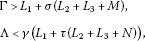where γ, ${L}_{i}$ ($i=1,2,3$) and σ, τ are defined by (2.4), (3.1) and (3.2), respectively, $M\ge 0$, $N\ge 0$, and

(${S}_{1}$) $f\left(t,u\right)\le \frac{c}{\mathrm{\Gamma }}$, for $\left(t,u\right)\in J×\left[0,c\right]$, and

$\sum _{i=1}^{j}{\rho }^{-1}p\left({t}_{i}\right)\varphi \left({t}_{i}\right){I}_{i}\left({u}_{i}\right)+\sum _{i=j+1}^{m}{\rho }^{-1}p\left({t}_{i}\right)\psi \left({t}_{i}\right){I}_{i}\left({u}_{i}\right)\le \frac{M}{\mathrm{\Gamma }}c,$

for ${u}_{i}\in \left[0,c\right]$, $i=0,1,\dots ,m$.

(${S}_{2}$) $f\left(t,u\right)<\frac{a}{\mathrm{\Gamma }}$, for $\left(t,u\right)\in J×\left[0,a\right]$, and

$\sum _{i=1}^{j}{\rho }^{-1}p\left({t}_{i}\right)\varphi \left({t}_{i}\right){I}_{i}\left({u}_{i}\right)+\sum _{i=j+1}^{m}{\rho }^{-1}p\left({t}_{i}\right)\psi \left({t}_{i}\right){I}_{i}\left({u}_{i}\right)\le \frac{M}{\mathrm{\Gamma }}a,$

for ${u}_{i}\in \left[0,a\right]$, $i=0,1,\dots ,m$.

(${S}_{3}$) $f\left(t,u\right)\ge \frac{b}{\mathrm{\Lambda }}$, for $\left(t,u\right)\in \left[\xi ,\eta \right]×\left[b,c\right]$, and

$\sum _{i=1}^{j}{\rho }^{-1}p\left({t}_{i}\right)\varphi \left({t}_{i}\right){I}_{i}\left({u}_{i}\right)+\sum _{i=j+1}^{m}{\rho }^{-1}p\left({t}_{i}\right)\psi \left({t}_{i}\right){I}_{i}\left({u}_{i}\right)\ge \frac{N}{\mathrm{\Lambda }}b,$

for $b\le {u}_{i}\le c$, $i=0,1,\dots ,m$.

Then IBVP (1.1) has at least three positive solutions ${x}_{1}$, ${x}_{2}$ and ${x}_{3}$ with

${\parallel {x}_{1}\parallel }_{PC}

and

$a<{\parallel {x}_{3}\parallel }_{PC},\phantom{\rule{2em}{0ex}}\underset{\xi \le t\le \eta }{min}|{x}_{3}\left(t\right)|

Proof We shall show that all the conditions of Lemma 2.6 are satisfied.

First, if $x\in {\overline{K}}_{c}$, then ${\parallel x\parallel }_{PC}\le c$ and (3.4), (3.6), (3.7) are valid if ${r}_{1}$ is replaced by $\frac{c}{\mathrm{\Gamma }}$. From this and (3.5), (3.8) and (3.9), for any $x\in {\overline{K}}_{c}$, we have

$\begin{array}{rcl}|Sx\left(t\right)|& =& {k}^{-1}\left({k}_{3}\varphi \left(t\right)+{k}_{4}\psi \left(t\right)\right)\left(\alpha \left[Fx\right]+\alpha \left[Qx\right]\right)\\ +{k}^{-1}\left({k}_{1}\varphi \left(t\right)+{k}_{2}\psi \left(t\right)\right)\left(\beta \left[Fx\right]+\beta \left[Qx\right]\right)+Fx\left(t\right)+Qx\left(t\right)\\ \le & {k}^{-1}\left({k}_{3}+{k}_{4}\right)\left(\alpha \left[Fx\right]+\alpha \left[Qx\right]\right)+{k}^{-1}\left({k}_{1}+{k}_{2}\right)\left(\beta \left[Fx\right]+\beta \left[Qx\right]\right)\\ +\underset{t\in J}{max}|Fx\left(t\right)|+\underset{t\in J}{max}|Qx\left(t\right)|\\ \le & {k}^{-1}\left({k}_{3}+{k}_{4}\right)\alpha \left[Fx\right]+{k}^{-1}\left({k}_{1}+{k}_{2}\right)\beta \left[Fx\right]+\underset{t\in J}{max}|Fx\left(t\right)|\\ +{k}^{-1}\left({k}_{3}+{k}_{4}\right)\left(\left(1-{k}_{1}\right)\sum _{i=1}^{j}{\rho }^{-1}p\left({t}_{i}\right)\varphi \left({t}_{i}\right){I}_{i}\left(x\left({t}_{i}\right)\right)\\ +{k}_{2}\sum _{i=j+1}^{m}{\rho }^{-1}p\left({t}_{i}\right)\psi \left({t}_{i}\right){I}_{i}\left(x\left({t}_{i}\right)\right)\right)\\ +{k}^{-1}\left({k}_{1}+{k}_{2}\right)\left({k}_{3}\sum _{i=1}^{j}{\rho }^{-1}p\left({t}_{i}\right)\varphi \left({t}_{i}\right){I}_{i}\left(x\left({t}_{i}\right)\right)\\ +\left(1-{k}_{4}\right)\sum _{i=j+1}^{m}{\rho }^{-1}p\left({t}_{i}\right)\psi \left({t}_{i}\right){I}_{i}\left(x\left({t}_{i}\right)\right)\right)\\ +\sum _{i=1}^{j}{\rho }^{-1}p\left({t}_{i}\right)\varphi \left({t}_{i}\right){I}_{i}\left(x\left({t}_{i}\right)\right)+\sum _{i=j+1}^{m}{\rho }^{-1}p\left({t}_{i}\right)\psi \left({t}_{i}\right){I}_{i}\left(x\left({t}_{i}\right)\right)\\ \le & {k}^{-1}\left({k}_{3}+{k}_{4}\right)\frac{{L}_{2}}{\mathrm{\Gamma }}c+{k}^{-1}\left({k}_{1}+{k}_{2}\right)\frac{{L}_{3}}{\mathrm{\Gamma }}c+\frac{{L}_{1}}{\mathrm{\Gamma }}c\\ +{k}^{-1}\left({k}_{3}+{k}_{4}\right)\sum _{i=1}^{j}{\rho }^{-1}p\left({t}_{i}\right)\varphi \left({t}_{i}\right){I}_{i}\left(x\left({t}_{i}\right)\right)\\ +{k}^{-1}\left({k}_{1}+{k}_{2}\right)\sum _{i=j+1}^{m}{\rho }^{-1}p\left({t}_{i}\right)\psi \left({t}_{i}\right){I}_{i}\left(x\left({t}_{i}\right)\right)\\ \le & \frac{1}{\mathrm{\Gamma }}\left[{L}_{1}+\sigma \left({L}_{2}+{L}_{3}+M\right)\right]c

which means that ${\parallel Sx\parallel }_{PC}\le c,x\in {\overline{K}}_{c}$. Therefore, $S:{\overline{K}}_{c}\to {\overline{K}}_{c}$. By Lemma 2.3, we know that $S:{\overline{K}}_{c}\to {\overline{K}}_{c}$ is completely continuous.

Next, it follows from condition (${S}_{2}$) that if $x\in {\overline{K}}_{a}$ then

$\begin{array}{rcl}{\parallel Sx\parallel }_{PC}& \le & {k}^{-1}\left({k}_{3}+{k}_{4}\right)\left(\alpha \left[Fx\right]+\alpha \left[Qx\right]\right)+{k}^{-1}\left({k}_{1}+{k}_{2}\right)\left(\beta \left[Fx\right]+\beta \left[Qx\right]\right)\\ +\underset{t\in J}{max}|Fx\left(t\right)|+\underset{t\in J}{max}|Qx\left(t\right)|\\ \le & \sigma \frac{{L}_{2}}{\mathrm{\Gamma }}a+\sigma \frac{{L}_{3}}{\mathrm{\Gamma }}a+\frac{{L}_{1}}{\mathrm{\Gamma }}a+\sigma \sum _{i=1}^{j}{\rho }^{-1}p\left({t}_{i}\right)\varphi \left({t}_{i}\right){I}_{i}\left(x\left({t}_{i}\right)\right)\\ +\sigma \sum _{i=j+1}^{m}{\rho }^{-1}p\left({t}_{i}\right)\psi \left({t}_{i}\right){I}_{i}\left(x\left({t}_{i}\right)\right)\\ \le & \frac{1}{\mathrm{\Gamma }}\left[{L}_{1}+\sigma \left({L}_{2}+{L}_{3}+M\right)\right]a

So the condition (${C}_{2}$) of Lemma 2.6 holds.

Now, we take $x\left(t\right)=\frac{b+c}{2}$, $t\in J$, then it is easy to see that $x\left(t\right)=\frac{b+c}{2}\in K\left(\phi ,b,c\right)$, and hence

$\phi \left(x\right)=\underset{\xi \le t\le \eta }{min}|x\left(t\right)|=\frac{b+c}{2}>b.$

Moreover, $\alpha \left[x\right]\ge 0$, $\beta \left[x\right]\ge 0$. This proves that $\left\{x\in K\left(\phi ,b,c\right):\phi \left(x\right)>b\right\}\ne \varphi$.

On the other hand, if $x\in K\left(\phi ,b,c\right)$, then $b\le x\left(t\right)\le c$, $t\in \left[\xi ,\eta \right]$. By condition (${S}_{3}$), we have

$\begin{array}{rcl}\phi \left(Sx\right)& =& \underset{\xi \le t\le \eta }{min}|Sx\left(t\right)|\\ =& \underset{\xi \le t\le \eta }{min}\left({k}^{-1}\left({k}_{3}\varphi \left(t\right)+{k}_{4}\psi \left(t\right)\right)\left(\alpha \left[Fx\right]+\alpha \left[Qx\right]\right)\\ +{k}^{-1}\left({k}_{1}\varphi \left(t\right)+{k}_{2}\psi \left(t\right)\right)\left(\beta \left[Fx\right]+\beta \left[Qx\right]\right)+Fx\left(t\right)+Qx\left(t\right)\right)\\ \ge & \gamma {k}^{-1}\left({k}_{3}+{k}_{4}\right)\left(\alpha \left[Fx\right]+\alpha \left[Qx\right]\right)+\gamma {k}^{-1}\left({k}_{1}+{k}_{2}\right)\left(\beta \left[Fx\right]+\beta \left[Qx\right]\right)\\ +\gamma {\int }_{0}^{1}G\left(s,s\right)p\left(s\right)h\left(s\right)f\left(s,x\left(s\right)\right)\phantom{\rule{0.2em}{0ex}}ds\\ +\gamma \sum _{i=1}^{j}{\rho }^{-1}p\left({t}_{i}\right)\varphi \left({t}_{i}\right){I}_{i}\left(x\left({t}_{i}\right)\right)+\gamma \sum _{i=j+1}^{m}{\rho }^{-1}p\left({t}_{i}\right)\psi \left({t}_{i}\right){I}_{i}\left(x\left({t}_{i}\right)\right)\\ \ge & \gamma \left\{{k}^{-1}\left({k}_{3}+{k}_{4}\right)\frac{{L}_{2}b}{\mathrm{\Lambda }}+{k}^{-1}\left({k}_{1}+{k}_{2}\right)\frac{{L}_{3}b}{\mathrm{\Lambda }}+\frac{{L}_{1}b}{\mathrm{\Lambda }}+{k}^{-1}\left({k}_{3}+{k}_{4}\right)\\ ×\left(\left(1-{k}_{1}\right)\sum _{i=1}^{j}{\rho }^{-1}p\left({t}_{i}\right)\varphi \left({t}_{i}\right){I}_{i}\left(x\left({t}_{i}\right)\right)+{k}_{2}\sum _{i=j+1}^{m}{\rho }^{-1}p\left({t}_{i}\right)\psi \left({t}_{i}\right){I}_{i}\left(x\left({t}_{i}\right)\right)\right)\\ +\sum _{i=1}^{j}{\rho }^{-1}p\left({t}_{i}\right)\varphi \left({t}_{i}\right){I}_{i}\left(x\left({t}_{i}\right)\right)+\sum _{i=j+1}^{m}{\rho }^{-1}p\left({t}_{i}\right)\psi \left({t}_{i}\right){I}_{i}\left(x\left({t}_{i}\right)\right)+{k}^{-1}\left({k}_{1}+{k}_{2}\right)\\ ×\left({k}_{3}\sum _{i=1}^{j}{\rho }^{-1}p\left({t}_{i}\right)\varphi \left({t}_{i}\right){I}_{i}\left(x\left({t}_{i}\right)\right)+\left(1-{k}_{4}\right)\sum _{i=j+1}^{m}{\rho }^{-1}p\left({t}_{i}\right)\psi \left({t}_{i}\right){I}_{i}\left(x\left({t}_{i}\right)\right)\right)\right\}\\ =& \gamma \left\{{k}^{-1}\left({k}_{3}+{k}_{4}\right)\frac{{L}_{2}b}{\mathrm{\Lambda }}+{k}^{-1}\left({k}_{1}+{k}_{2}\right)\frac{{L}_{3}b}{\mathrm{\Lambda }}+\frac{{L}_{1}b}{\mathrm{\Lambda }}+{k}^{-1}\left({k}_{3}+{k}_{4}\right)\\ ×\sum _{i=1}^{j}{\rho }^{-1}p\left({t}_{i}\right)\varphi \left({t}_{i}\right){I}_{i}\left(x\left({t}_{i}\right)\right)+{k}^{-1}\left({k}_{1}+{k}_{2}\right)\sum _{i=j+1}^{m}{\rho }^{-1}p\left({t}_{i}\right)\psi \left({t}_{i}\right){I}_{i}\left(x\left({t}_{i}\right)\right)\right\}\\ \ge & \frac{1}{\mathrm{\Lambda }}\gamma \left({L}_{1}+\tau \left({L}_{2}+{L}_{3}+N\right)\right)b\\ >& b,\end{array}$

which implies that $\phi \left(Sx\right)>b$, for $x\in K\left(\phi ,b,c\right)$. This shows that condition (${C}_{1}$) of Lemma 2.6 is also satisfied.

By Lemma 2.6 and Remark 2.3, IBVP (1.1) has at least three positive solutions ${x}_{1},{x}_{2}$ and ${x}_{3}$ such that

${\parallel {x}_{1}\parallel }_{PC}

and

$a<{\parallel {x}_{3}\parallel }_{PC},\phantom{\rule{2em}{0ex}}\underset{\xi \le t\le \eta }{min}|{x}_{3}\left(t\right)|

The proof of Theorem 3.3 is completed. □

## 4 Examples

Example 4.1 Consider the following singular IBVP

$\left\{\begin{array}{c}{x}^{″}\left(t\right)-x\left(t\right)+\frac{\sqrt{t\left(1-t\right)}}{\left({e}^{1+t}-{e}^{1-t}\right)\left({e}^{2-t}-{e}^{t}\right)}\left(\sqrt{{t}^{2}+1}|lnx|\right)=0,\phantom{\rule{1em}{0ex}}t\in \left(0,1\right)\setminus \left\{{t}_{1}\right\},\hfill \\ -\mathrm{△}{x}^{\prime }{|}_{t={t}_{1}}=\sqrt{x\left({t}_{1}\right)},\phantom{\rule{1em}{0ex}}{t}_{1}=\frac{1}{2},\hfill \\ x\left(0\right)=\alpha \left[x\right],\phantom{\rule{2em}{0ex}}x\left(1\right)=\beta \left[x\right].\hfill \end{array}$
(4.1)

We conclude that IBVP (4.1) has at least one positive solution.

Proof IBVP (4.1) can be regarded as a IBVP of the form (1.1), where $a\left(t\right)\equiv 0$, $b\left(t\right)\equiv -1$, $h\left(t\right)=\frac{\sqrt{t\left(1-t\right)}}{\left({e}^{1+t}-{e}^{1-t}\right)\left({e}^{2-t}-{e}^{t}\right)}$, $f\left(t,u\right)=\sqrt{{t}^{2}+1}|lnu|$, ${I}_{1}\left(u\right)=\sqrt{u}$. It is not difficult to see that $h\left(t\right)$ is singular at $t=0$ and $t=1$, $0<{\int }_{0}^{1}h\left(t\right)\phantom{\rule{0.2em}{0ex}}dt<+\mathrm{\infty }$, $h\left(t\right)\ge 0$ for $t\in \left(0,1\right)$, $f\left(t,u\right)\ge 0$, ${I}_{1}\left(u\right)\ge 0$ for $t\in J$, $u\in {\mathbb{R}}^{+}$. Choosing $\xi =\frac{1}{4}$, $\eta =\frac{3}{4}$, then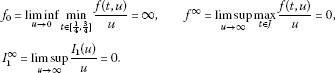Let ϕ and ψ satisfy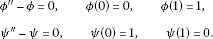Then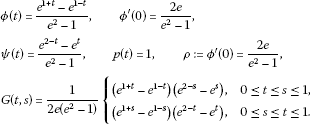Case 1. Let $\alpha \left[x\right]=\frac{{e}^{2}-1}{2\left({e}^{\frac{7}{4}}-{e}^{\frac{1}{4}}\right)}x\left(\frac{1}{4}\right)$, $\beta \left[x\right]=\frac{{e}^{2}-1}{2\left({e}^{\frac{7}{4}}-{e}^{\frac{1}{4}}\right)}x\left(\frac{3}{4}\right)$. By direct calculation, we have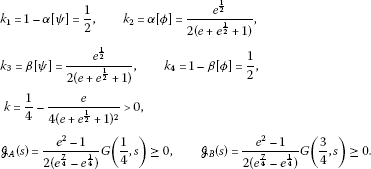So all the conditions of Theorem 3.2 are satisfied. By Theorem 3.2, IBVP (4.1) has at least one positive solution.

Case 2. Let $\alpha \left[x\right]={\int }_{0}^{1}x\left(t\right)\left(4{\pi }^{2}+1\right)cos2\pi t\phantom{\rule{0.2em}{0ex}}dt$, that is $dA\left(t\right)=\left(4{\pi }^{2}+1\right)cos2\pi t\phantom{\rule{0.2em}{0ex}}dt$, so the measure dA changes sign on $\left[0,1\right]$. For convenience, we still take $\beta \left[x\right]=\frac{{e}^{2}-1}{2\left({e}^{\frac{7}{4}}-{e}^{\frac{1}{4}}\right)}x\left(\frac{3}{4}\right)$. By direct calculation, we have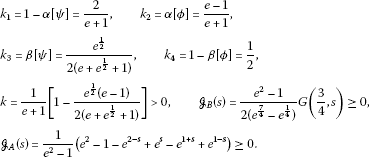So all conditions of Theorem 3.2 are satisfied. By Theorem 3.2, IBVP (4.1) has at least one positive solution. □

Example 4.2 Consider the following singular IBVP

$\left\{\begin{array}{c}{x}^{″}\left(t\right)+\frac{t}{1+t}{x}^{\prime }\left(t\right)-\frac{1}{1+t}x\left(t\right)+h\left(t\right)f\left(t,x\left(t\right)\right)=0,\phantom{\rule{1em}{0ex}}t\in \left(0,1\right)\setminus \left\{{t}_{1}\right\},\hfill \\ -\mathrm{△}{x}^{\prime }{|}_{t={t}_{1}}={I}_{1},\phantom{\rule{1em}{0ex}}{t}_{1}=\frac{1}{2},\hfill \\ x\left(0\right)=x\left(\frac{1}{2}\right),\phantom{\rule{2em}{0ex}}x\left(1\right)=\frac{1}{4}x\left(\frac{1}{2}\right),\hfill \end{array}$
(4.2)

where

$h\left(t\right)=\left\{\begin{array}{cc}\frac{1+t}{\left(2{e}^{-\frac{1}{2}}-{e}^{-1}\right)t\sqrt{t}{e}^{t}},\hfill & 0\le t\le \frac{1}{2},\hfill \\ \frac{1+t}{\left(1-t{e}^{t-1}\right)\sqrt{1-t}},\hfill & \frac{1}{2}\le t\le 1\hfill \end{array}$

and

$f\left(t,u\right)=\left\{\begin{array}{cc}\frac{1}{50}\left(t+1\right)\left(10-u\right),\hfill & 0\le t\le 1,0\le u\le 10,\hfill \\ \frac{1}{5}\left(t+1\right){\left(u-10\right)}^{2},\hfill & 0\le t\le 1,10\le u\le 20,\hfill \\ \frac{1}{196}\left(t+1\right)\left(u+3900\right),\hfill & 0\le t\le 1,u\ge 20.\hfill \end{array}$

We conclude that IBVP (4.2) has at least three positive solutions.

Proof IBVP (4.2) can be regarded as a IBVP of the form (1.1), where $a\left(t\right)=\frac{t}{1+t}$, $b\left(t\right)=-\frac{1}{1+t}$. It is not difficult to see that $h\left(t\right)$ is singular at $t=0$ and $t=1$, $0<{\int }_{0}^{1}h\left(t\right)\phantom{\rule{0.2em}{0ex}}dt<+\mathrm{\infty }$, $h\left(t\right)\ge 0$ for $t\in \left(0,1\right)$, $f\left(t,u\right)\ge 0$ for $t\in J$, $u\in {\mathbb{R}}^{+}$.

Let ϕ and ψ satisfy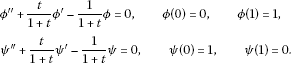Then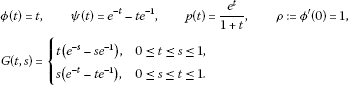By direct calculation, we have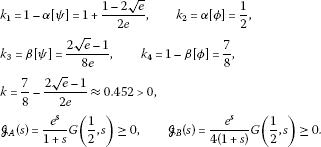Therefore the conditions (${H}_{1}$)-(${H}_{3}$) hold. In addition, $\sigma =\frac{12e+4-8\sqrt{e}}{7e+4-8\sqrt{e}}$, $\tau =\frac{7e-1+2\sqrt{e}}{7e+4-8\sqrt{e}}$,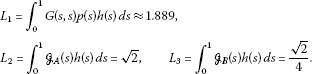Let $\xi =\frac{1}{8}$, $\eta =\frac{3}{4}$, $M=5$, $N=0$. Then $\gamma =\frac{1}{8}$, $\mathrm{\Gamma }>18.002$, $0<\mathrm{\Lambda }<0.715$. Take $\mathrm{\Gamma }=20$, $\mathrm{\Lambda }=\frac{1}{2}$, $a=10$, $b=20$, $c=1000$. Then $0<{I}_{1}\le 4.545$. Consequently, all the assumptions of Theorem 3.3 are satisfied, and thus, by Theorem 3.3, we infer that the singular IBVP (4.2) has at least three positive solutions ${x}_{1}$, ${x}_{2}$ and ${x}_{3}$ satisfying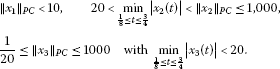□

## References

1. Bainov DD, Simeonov PS: Systems with Impulse Effect. Ellis Horwood, Chichester; 1989.

2. Lakshmikantham V, Bainov DD, Simeonov PS: Theory of Impulsive Differential Equations. World Scientific, Singapore; 1989.

3. Agarwal RP, O’Regan D: Multiple nonnegative solutions for second order impulsive differential equations. Appl. Math. Comput. 2000, 114: 51–59. 10.1016/S0096-3003(99)00074-0

4. Agarwal RP, O’Regan D: A multiplicity result for second order impulsive differential equations via the Leggett Williams fixed point theorem. Appl. Math. Comput. 2005, 161: 433–439. 10.1016/j.amc.2003.12.096

5. Hao X, Liu L, Wu Y: Positive solutions for second order impulsive differential equations with integral boundary conditions. Commun. Nonlinear Sci. Numer. Simul. 2011, 16: 101–111. 10.1016/j.cnsns.2010.04.007

6. Jankowski T: Positive solutions to second order four-point boundary value problems for impulsive differential equations. Appl. Math. Comput. 2008, 202: 550–561. 10.1016/j.amc.2008.02.040

7. Jankowski T: Positive solutions for second order impulsive differential equations involving Stieltjes integral conditions. Nonlinear Anal. 2011, 74: 3775–3785. 10.1016/j.na.2011.03.022

8. Lee EK, Lee YH: Multiple positive solutions of singular two point boundary value problems for second order impulsive differential equations. Appl. Math. Comput. 2004, 158: 745–759. 10.1016/j.amc.2003.10.013

9. Lin X, Jiang D: Multiple positive solutions of Dirichlet boundary value problems for second order impulsive differential equations. J. Math. Anal. Appl. 2006, 321: 501–514. 10.1016/j.jmaa.2005.07.076

10. Feng M, Xie D: Multiple positive solutions of multi-point boundary value problem for second-order impulsive differential equations. J. Comput. Appl. Math. 2009, 223: 438–448. 10.1016/j.cam.2008.01.024

11. Li J, Shen J: Multiple positive solutions for a second-order three-point boundary value problem. Appl. Math. Comput. 2006, 182: 258–268. 10.1016/j.amc.2006.01.095

12. Liu B, Liu L, Wu Y: Positive solutions for a singular second-order three-point boundary value problem. Appl. Math. Comput. 2008, 196: 532–541. 10.1016/j.amc.2007.06.013

13. Ma R, Ren L: Positive solutions for nonlinear m -point boundary value problems of Dirichlet type via fixed-point index theory. Appl. Math. Lett. 2003, 16: 863–869. 10.1016/S0893-9659(03)90009-7

14. Ma R, Wang H: Positive solutions of nonlinear three-point boundary-value problems. J. Math. Anal. Appl. 2003, 279: 216–227. 10.1016/S0022-247X(02)00661-3

15. Sun J, Li W, Zhao Y: Three positive solutions of a nonlinear three-point boundary value problem. J. Math. Anal. Appl. 2003, 288: 708–716. 10.1016/j.jmaa.2003.09.019

16. Hu L, Liu L, Wu Y: Positive solutions of nonlinear singular two-point boundary value problems for second-order impulsive differential equations. Appl. Math. Comput. 2008, 196: 550–562. 10.1016/j.amc.2007.06.014

17. Guo D, Lakshmikantham V: Nonlinear Problems in Abstract Cone. Academic Press, New York; 1988.

18. Guo D, Sun J, Liu Z: Functional Method for Nonlinear Ordinary Differential Equation. Shandong Science and Technology Press, Jinan; 1995. (in Chinese)

19. Leggett RW, Williams LR: Multiple positive fixed points of nonlinear operators on ordered Banach space. Indiana Univ. Math. J. 1979, 28: 673–688. 10.1512/iumj.1979.28.28046

## Acknowledgements

The authors thank the referee for helpful comments and suggestions, which lead to an improvement of the paper. The first and second authors were supported financially by the National Natural Science Foundation of China (11071141, 11126231) and the Natural Science Foundation of Shandong Province of China (ZR2010AM017, ZR2011AQ008). The third author was supported financially by the Australia Research Council through an ARC Discovery Project Grant.

## Author information

Authors

### Corresponding author

Correspondence to Lishan Liu.

### Competing interests

The authors declare that they have no competing interests.

### Authors’ contributions

The study was carried out in collaboration between all authors. JJ completed the main part of this paper and gave two examples; LL and YW corrected the main theorems and polished the manuscript. All authors read and approved the final manuscript.

## Rights and permissions

Reprints and Permissions

Jiang, J., Liu, L. & Wu, Y. Positive solutions for second order impulsive differential equations with Stieltjes integral boundary conditions. Adv Differ Equ 2012, 124 (2012). https://doi.org/10.1186/1687-1847-2012-124

• Accepted:

• Published:

• DOI: https://doi.org/10.1186/1687-1847-2012-124

### Keywords

• impulsive differential equation
• positive solutions
• fixed point theorem
• integral boundary conditions